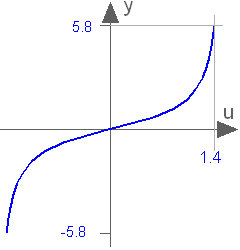# 'tan()'

tan()# Information

This information is part of the Modelica Standard Library maintained by the Modelica Association.

Trigonometric tangent function

`tan(u)`

#### Description

Returns the tangent of u, with -∞ < u < ∞ (if u is a multiple of (2n-1)*pi/2, y = tan(u) is +/- infinity). Argument u needs to be an Integer or Real expression.

The tangent function can also be accessed as Modelica.Math.tan.#### Examples

```tan(3.14159265358979)
= 0.0
```